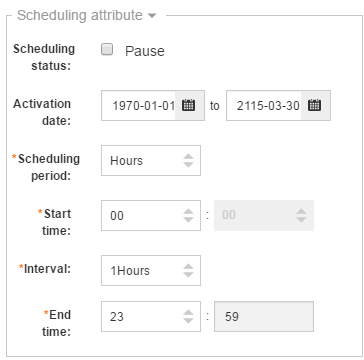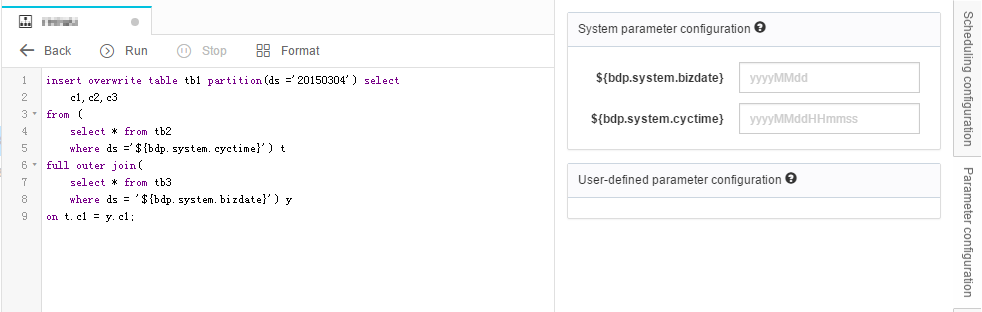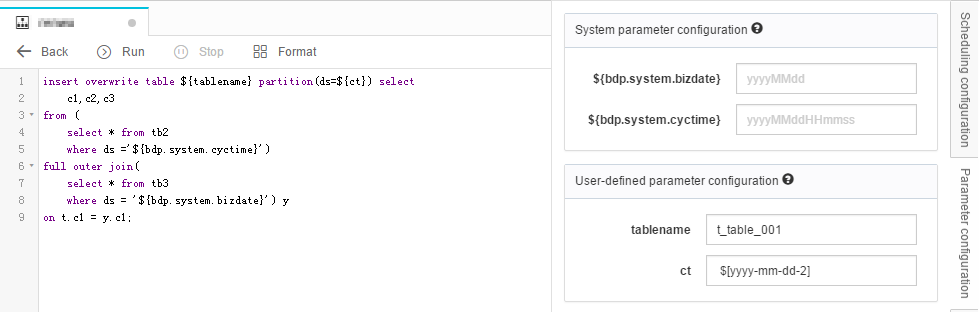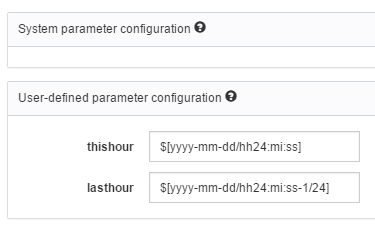All Products
Search
Document Center

# System scheduling parameters

Last Updated: Apr 25, 2018

For scheduling tasks to adapt to the network environment changes, DataWorks provides the function of the parameter configuration, as shown in the following table.

Parameter type Setting Applicable type Example
System parameters: bdp.system.bizdate and bdp.system.cyctime When they are running in the scheduling system, configuration can be skipped, and they can be called directly as \${bdp.system.bizdate} and \${bdp.system.cyctime} in code. The system automatically replaces their values. All node type -
User-defined parameters The user-defined parameters can be called as \${key1} and \${key2} in code, and then you can configure them by using “key1=value1, key2=value2”. Other node types except SHELL Constant parameters: param1=”abc”, param2=1234; variable parameters: param1=\$[yyyymmdd], and the result is calculated based on the value of bdp.system.cyctime.
User-defined parameters The user-defined parameters can be called as \$1, \$2, and \$3 in code, and then you can configure them by using value1, value2, and value3. SHELL type Constant parameter: ”abc” 1234; variable parameter: \$[yyyymmdd], and the result is calculated based on the value of bdp.system.cyctime.

## System parameter

Currently, only two system scheduling parameters are available, which are used only in the code and you do not need to assign values to them. Their values get automatically replaced during automatic node scheduling. The two system parameters \${bdp.system.bizdate} and \${bdp.system.cyctime} are described as follows.

\${bdp.system.bizdate}

Format: yyyymmdd. The previous day’s (Year Month Date) scheduled time of the daily scheduled instance; and is equal to the time (year-month-day) for the instance of the automatically scheduled daily node, minus 1 day.

Note:
In normal scheduling, for example: the job is scheduled to run early morning of the 14th, \${bdp.system.bizdate} is replaced by the value of the 13th.

During testing or value population, for example: if the operational date is selected to be the 12th, \${bdp.system.bizdate} is replaced by the value of the 12th.

\${bdp.system.cyctime}

This format is: yyyymmddhh24miss. It specifies the scheduled time (Year Month Date Hour Minute Second) for the routinely scheduled instance.

Item Description
yyyy A four-digit year
mm A two-digit month
dd A two-digit day
hh24 The time in the 24-hour format
mi A two-digit time in minutes
ss A two-digit time in seconds

Note:
In normal scheduling, for example: The job is scheduled to run 01:00 on the 14th, \${bdp.system.cyctime} is replaced by yyyymm140100 of that day.

During testing or value population, for example, if the business date is selected to be the 12th (the job scheduling time is configured to be at 01:00 of every day), \${bdp.system.cyctime} is replaced by the yyyymm130100 of the selected business date.

For example, configure the workflow to be scheduled by hour, to run every other hour from 00:00 to 23:59 every day.

The node code is as follows.

``insert overwrite table tb1 partition(ds ='20150304') select``    c1,c2,c3``from (``    select * from tb2``    where ds ='\${bdp.system.cyctime}') t``full outer join(``    select * from tb3``    where ds = '\${bdp.system.bizdate}') y``on t.c1 = y.c1;``

Parameter attribute configuration of the corresponding workflow and node is as follows.The workflow scheduled to generate 24 instances on October 27, 2015.

1) The first instance was scheduled at 00:00:00 on 2015-10-27, then:

bdp.system.cyctime is replaced by 20151027000000;

bdp.system.bizdate is replaced by 20151026.

2) The second instance was scheduled at 1:00:00 AM on 2015-10-27, then:

bdp.system.cyctime is replaced by 20151027010000;

bdp.system.bizdate is replaced by 20151026.

3) Following the same rule, the 24th instance was scheduled at 23:00:00 on 2015-10-27, then:

bdp.system.cyctime is replaced by 20151027230000;

bdp.system.bizdate is replaced by 20151026.

## User-defined parameters

### Declaring variables: \${variable name}

User-defined parameters support user-defined variable names in the code. You must assign values to the user-defined variables during scheduling. In the DataWorks Kit, variables are declared in the form of \${variable name}. You can assign values to the parameter following the variable name=scheduling parameter or constant rule.

For example, configure the table name to be inserted in the node code into the \${tablename} variable.

The node code is as follows.

``insert overwrite table \${tablename} partition(ds =\${ct}) select``    c1,c2,c3``from (``    select * from tb2``    where ds ='\${bdp.system.cyctime}') t``full outer join(``    select * from tb3``    where ds = '\${bdp.system.bizdate}') y``on t.c1 = y.c1;``

Parameter attribute configuration of the corresponding node is shown in the following figure.When the node is scheduled to run, \${tablename} in the code is replaced by ‘t_table_001’, \${ct} in the code is replaced by ‘\$[yyyy-mm-dd-2]’. It represents data obtained two days before the date of service.

### The format of \$[…] is customized based on the system parameter “\${bdp.system.cyctime}” .

In \${bdp.system.cyctime}=\$[yyyymmddhh24miss], you can freely separate or combine the elements or specify the format. For example, you can define the year-month-day format of the scheduled time as \$[yyyymmdd] or \$[yyyy-mm-dd], and define the hour-minute-second format of the scheduled time as \$[hh24miss] or \$[hh24:mi:ss].

Note:
No space is allowed in any of the parameters. For detailed usage with the cyctime as the benchmark for running, see the following instructional documents. The time calculation method is the same as that of Oracle. During data population, the parameter value after replacement is the business date + 1 day. For example, if the date of 20140510 is selected as the business date, the cyctime is replaced by 20140511.

How to calculate the date after +/- a certain number of cycles are shown as follows.

• N years later: \$[add_months(yyyymmdd,12*N)]

• N years earlier: \$[add_months(yyyymmdd,-12*N)]

• N months later: \$[add_months(yyyymmdd,N)]

• N months earlier: \$[add_months(yyyymmdd,-N)]

• N weeks later: \$[yyyymmdd+7*N]

• N weeks earlier: \$[yyyymmdd-7*N]

• N days later: \$[yyyymmdd+N]

• N days earlier: \$[yyyymmdd-N]

• N hours later: \$[hh24miss+N/24]

• N hours earlier: \$[hh24miss-N/24]

• N minutes later: \$[hh24miss+N/24/60]

• N minutes earlier: \$[hh24miss-N/24/60]

For example, configure the workflow to be scheduled by hour, and to be run every other hour from 00:00 to 23:59 every day. The MaxCompute SQL node code is as follows.

``insert overwrite table tb1 partition(ds ='20150304') select``    c1,c2,c3``from (``    select * from tb2``    where ds ='\${thishour}') t``full outer join(``    select * from tb3``    where ds = '\${lasthour}') y``on t.c1 = y.c1;``

The workflow and node attributes are shown as follows.Configuration of user-defined parameters is shown as follows.

thishour=\$[yyyy-mm-dd/hh24:mi:ss]

lasthour =\$[yyyy-mm-dd/hh24:mi:ss-1/24]

The workflow generated 24 instances on October 27, 2015.

• 1) Because the scheduled time for the first instance is 2015-10-27 00:00:00, “thishour” is replaced by 2015-10-27/00:00:00, and “lasthour” is replaced by 2015-10-26/23:00:00.

• 2) Because the scheduled time for the second instance is 2015-10-27 01:00:00, “thishour” is replaced by 2015-10-27/01:00:00, and “lasthour” is replaced by 2015-10-27/00:00:00.

• 3) Similarly, the scheduled time for the 24th instance is 2015-10-27 23:00:00, “thishour” is replaced by 2015-10-27/23:00:00, and “lasthour” is replaced by 2015-10-27/22:00:00.

## FAQ

The table partition format is: pt=yyyy-mm-dd hh24:mi:ss, but the \$[yyyy-mm-dd hh24:mi:ss] result is yyyy-mm-ddhh24:mi:ss. How do I resolve this?

The code must read pt=”\${datetime} \${hour}” (note the space between the two variables) and assign the value of datetime=\$[yyyy-mm-dd] and hour=\$[hh24:mi:ss] to parameters in node configuration.

The table partition format is: day=yyyymmdd, hour=hh24, and the data in the previous hour is retrieved for any execution. The \$[yyyymmdd] \$[hh24-1/24] can be met, but it changes to 23:00 of the day at 00:00. How do I resolve this?

The A: code must read day=\${datetime}, hour=\${hour}, and assign the value of datetime=\$[yyyymmdd-1/24] and hour=\$[hh24-1/24] to parameters in node configuration.